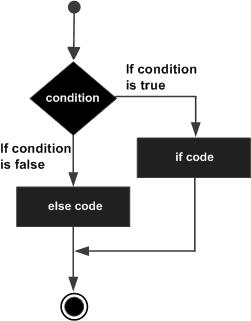# Perl IF...ELSE statement

A Perl if statement can be followed by an optional else statement, which executes when the boolean expression is false.

## Syntax

The syntax of an if...else statement in Perl programming language is −

```if(boolean_expression) {
# statement(s) will execute if the given condition is true
} else {
# statement(s) will execute if the given condition is false
}
```

If the boolean expression evaluates to true, then the if block of code will be executed otherwise else block of code will be executed.

The number 0, the strings '0' and "" , the empty list () , and undef are all false in a boolean context and all other values are true. Negation of a true value by ! or not returns a special false value.

## Flow Diagram## Example

```#!/usr/local/bin/perl

\$a = 100;
# check the boolean condition using if statement
if( \$a < 20 ) {
# if condition is true then print the following
printf "a is less than 20\n";
} else {
# if condition is false then print the following
printf "a is greater than 20\n";
}
print "value of a is : \$a\n";

\$a = "";
# check the boolean condition using if statement
if( \$a ) {
# if condition is true then print the following
printf "a has a true value\n";
} else {
# if condition is false then print the following
printf "a has a false value\n";
}
print "value of a is : \$a\n";
```

When the above code is executed, it produces the following result −

```a is greater than 20
value of a is : 100
a has a false value
value of a is :
```
perl_conditions.htm